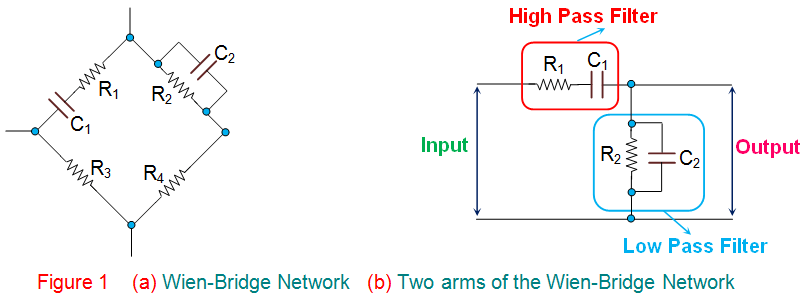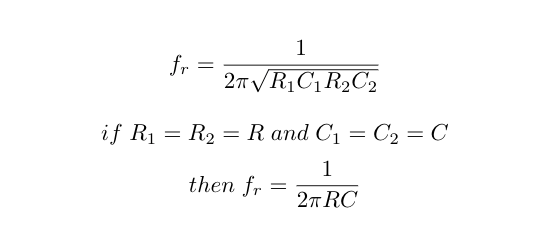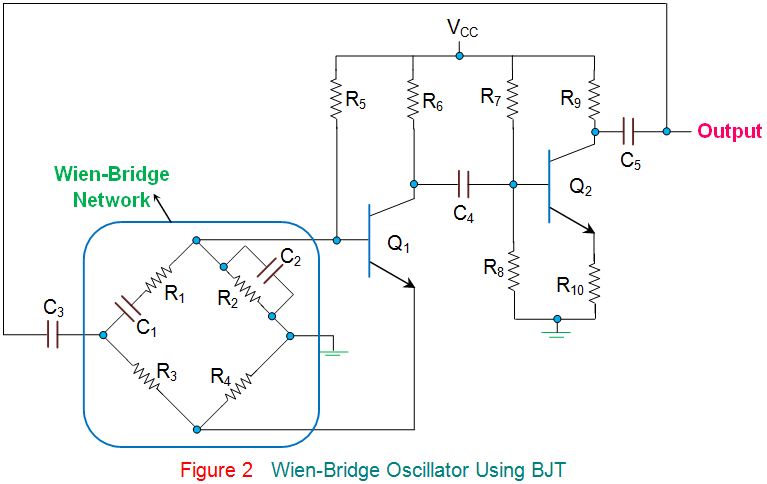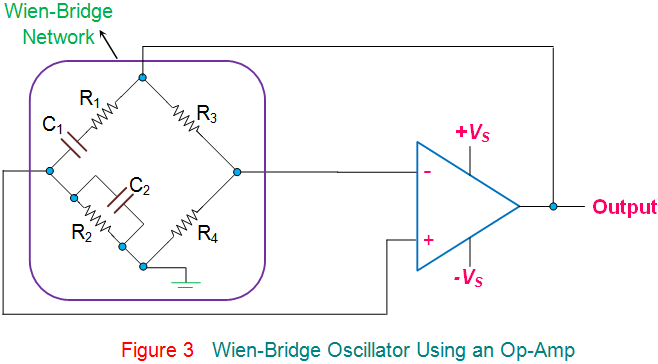# Wien Bridge Oscillator: Circuit And Frequency Calculation

## What is a Wien Bridge Oscillator?

A Wien-Bridge Oscillator is a type of phase-shift oscillator which is based upon a Wien-Bridge network (Figure 1a) comprising of four arms connected in a bridge fashion. Here two arms are purely resistive while the other two arms are a combination of resistors and capacitors.

In particular, one arm has resistor and capacitor connected in series (R1 and C1) while the other has them in parallel (R2 and C2).

This indicates that these two arms of the network behave identical to that of high pass filter or low pass filter, mimicking the behavior of the circuit shown by Figure 1b.In this circuit, at high frequencies, the reactance of the capacitors C1 and C2 will be much less due to which the voltage V0 will become zero as R2 will be shorted.

Next, at low frequencies, the reactance of the capacitors C1 and C2 will become very high.

However even in this case, the output voltage V0 will remain at zero only, as the capacitor C1 would be acting as an open circuit.

This kind of behavior exhibited by the Wien-Bridge network makes it a lead-lag circuit in the case of low and high frequencies, respectively.

## Wien Bridge Oscillator Frequency Calculation

Nevertheless, amidst these two high and low frequencies, there exists a particular frequency at which the values of the resistance and the capacitive reactance will become equal to each other, producing the maximum output voltage.

This frequency is referred to as resonant frequency. The resonant frequency for a Wein Bridge Oscillator is calculated using the following formula:Further, at this frequency, the phase-shift between the input and the output will become zero and the magnitude of the output voltage will become equal to one-third of the input value. In addition, it is seen that the Wien-Bridge will be balanced only at this particular frequency.

In the case of Wien-Bridge oscillator, the Wien-Bridge network of Figure 1 will be used in the feedback path as shown in Figure 2. The circuit diagram for a Wein Oscillator using a BJT (Bipolar Junction Transistor) is shown below:In these oscillators, the amplifier section will comprise of two-stage amplifier formed by the transistors, Q1 and Q2, wherein the output of Q2 is back-fed as an input to Q1 via Wien-Bridge network (shown within the blue enclosure in the figure).

Here, the noise inherent in the circuit will cause a change in the base current of Q1 which will appear at its collector point after being amplified with a phase-shift of 180o.

This is fed as an input to Q2 via C4 and gets further amplified and appears with an additional phase-shift of 180o.

This makes the net phase-difference of the signal fed back to the Wien-Bridge network to be 360o, satisfying phase-shift criterion to obtain sustained oscillations.

However, this condition will be satisfied only in the case of resonant frequency, due to which the Wien-Bridge oscillators will be highly selective in terms of frequency, leading to a frequency-stabilized design.

Wien-bridge oscillators can even be designed using Op-Amps as a part of their amplifier section, as shown by Figure 3.

However it is to be noted that, here, the Op-Amp is required to act as a non-inverting amplifier as the Wien-Bridge network offers zero phase-shift.

Further, from the circuit, it is evident that the output voltage is fed back to both inverting and non-inverting input terminals.

At resonant frequency, the voltages applied to the inverting and non-inverting terminals will be equal and in-phase with each other.

However, even here, the voltage gain of the amplifier needs to be greater than 3 to start oscillations and equal to 3 to sustain them. In general, these kind of Op-Amp-based Wien Bridge Oscillators cannot operate above 1 MHz due to the limitations imposed on them by their open-loop gain.Wien-Bridge networks are low frequency oscillators which are used to generate audio and sub-audio frequencies ranging between 20 Hz to 20 KHz.

Further, they provide stabilized, low distorted sinusoidal output over a wide range of frequency which can be selected using decade resistance boxes.

In addition, the oscillation frequency in this kind of circuit can be varied quite easily as it just needs variation of the capacitors C1 and C2.

However these oscillators require large number of circuit components and can be operated upto a certain maximum frequency only.

Want To Learn Faster? 🎓
Get electrical articles delivered to your inbox every week.
No credit card required—it’s 100% free.Electrical4U is dedicated to the teaching and sharing of all things related to electrical and electronics engineering.

### 1 thought on “Wien Bridge Oscillator: Circuit And Frequency Calculation”

1.hi!